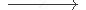Share

# Correct and Balance the Following Equations: N + H Nh3 - CBSE Class 10 - Science

#### Question

Correct and balance the following equation:

N    +   HNH3

#### Solution

N2 + 3H2 → 2NH3

Is there an error in this question or solution?

#### APPEARS IN

Lakhmir Singh Solution for Chemistry for Class 10 (2019 Exam) (2018 to Current)
Chapter 1: Chemical Reactions and Equations
Q: 13.2 | Page no. 19

#### Video TutorialsVIEW ALL 

Solution Correct and Balance the Following Equations: N + H Nh3 Concept: Balanced Chemical Equation.
S